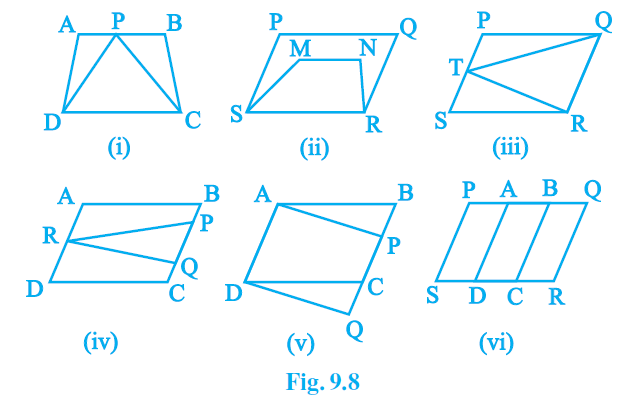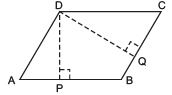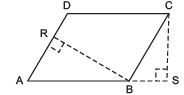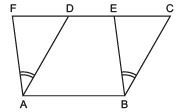UPSC  >  Exercise 9.1 NCERT Solutions - Areas of Parallelograms and Triangles

# Exercise 9.1 NCERT Solutions - Areas of Parallelograms and Triangles - NCERT Textbooks (Class 6 to Class 12) - UPSC

Q1. Which of the following figures lie on the same base and between the same parallels.
In such a case, write the common base and the two parallels.Ans: The figures (i), (iii), and (v) lie on the same base and between the same parallels.

 Common base Two parallels Fig. (i)Fig. (iii)Fig. (v) DCQRAD DC and ABQR and PSAD and BQ

## Parallelograms on the same Base and between the same Parallels

Let us recall that “A diagonal of a parallelogram divides it into two triangles of equal area.” In case of a parallelogram, any side can be called its base, whereas the length of the line segment which is perpendicular to the base from the opposite side is called the altitude or height of the parallelogram corresponding to the given base.
In the figure,

• DP is the altitude of parallelogram ABCD, corresponding to the base AB.• DQ is the altitude of parallelogram ABCD, corresponding to the base BC.
• BR is the altitude of parallelogram ABCD, corresponding to the base AD.
• CS is the altitude of parallelogram ABCD, corresponding to the base AB.

### Theorem

Parallelograms on the same base (or on equal bases) and between the same parallels are equal in area.

### Proof

Let us have two parallelogram ABCD and ABEF on the same base AB and between the same parallel lines AB and FC.In ΔADF and ΔBCE, AD = BC      [Opposite sides of a parallelogram]
AF = BE                          [Opposite sides of a parallelogram]
∠DAF = ∠CBE                 [Since AD || BC and AF || BE]
∴ ΔADF ≌ ΔBCE [SAS criteria]But congruent triangles are equal in area. ∴
ar (ΔADF) = ar (ΔBCE) Adding ar (ABED) to both sides, we have
ar (ΔADF) + ar (ABED) + ar (BCE) + ar (ABED)
⇒ ar (parallelogram ABEF) = ar (parallelogram ABCD).

Remember

• Area of a parallelogram is the product of its any side and the corresponding altitude.
• Parallelograms on the same base (or equal bases) and between the same parallels are equal in area.
• Parallelograms on the same base (or equal bases) and having equal areas lie between the same parallels.
The document Exercise 9.1 NCERT Solutions - Areas of Parallelograms and Triangles | NCERT Textbooks (Class 6 to Class 12) - UPSC is a part of the UPSC Course NCERT Textbooks (Class 6 to Class 12).
All you need of UPSC at this link: UPSC

## NCERT Textbooks (Class 6 to Class 12)

1402 docs|679 tests

## FAQs on Exercise 9.1 NCERT Solutions - Areas of Parallelograms and Triangles - NCERT Textbooks (Class 6 to Class 12) - UPSC

 1. What are the properties of parallelograms on the same base and between the same parallels?Ans. Parallelograms on the same base and between the same parallels have the following properties: - They have the same base length. - They lie between the same parallel lines. - They have equal heights. - They have equal areas.
 2. How can I find the area of parallelograms on the same base and between the same parallels?Ans. To find the area of parallelograms on the same base and between the same parallels, you can use the formula: Area = Base * Height. Since these parallelograms have the same base and height, their areas will be equal.
 3. Can the area of parallelograms on the same base and between the same parallels be negative?Ans. No, the area of parallelograms cannot be negative. Area is a measure of the space enclosed by a shape, and it is always a positive value or zero. Therefore, the area of parallelograms on the same base and between the same parallels will always be a non-negative value.
 4. What is the significance of parallelograms on the same base and between the same parallels?Ans. Parallelograms on the same base and between the same parallels have equal areas. This property is useful in various mathematical and real-life applications. For example, it can be used to calculate the area of irregular shapes by dividing them into parallelograms with the same base and between the same parallels.
 5. Can parallelograms on the same base and between the same parallels have different shapes?Ans. Yes, parallelograms on the same base and between the same parallels can have different shapes. As long as they have the same base length and lie between the same parallel lines, they can have different angles and side lengths. The only requirement for them to be considered parallelograms on the same base and between the same parallels is that their bases are equal in length and they lie between the same parallel lines.

## NCERT Textbooks (Class 6 to Class 12)

1402 docs|679 testsExplore Courses for UPSC exam### How to Prepare for UPSC

Read our guide to prepare for UPSC which is created by Toppers & the best Teachers
Signup to see your scores go up within 7 days! Learn & Practice with 1000+ FREE Notes, Videos & Tests.
10M+ students study on EduRev
Track your progress, build streaks, highlight & save important lessons and more!
Related Searches

,

,

,

,

,

,

,

,

,

,

,

,

,

,

,

,

,

,

,

,

,

;